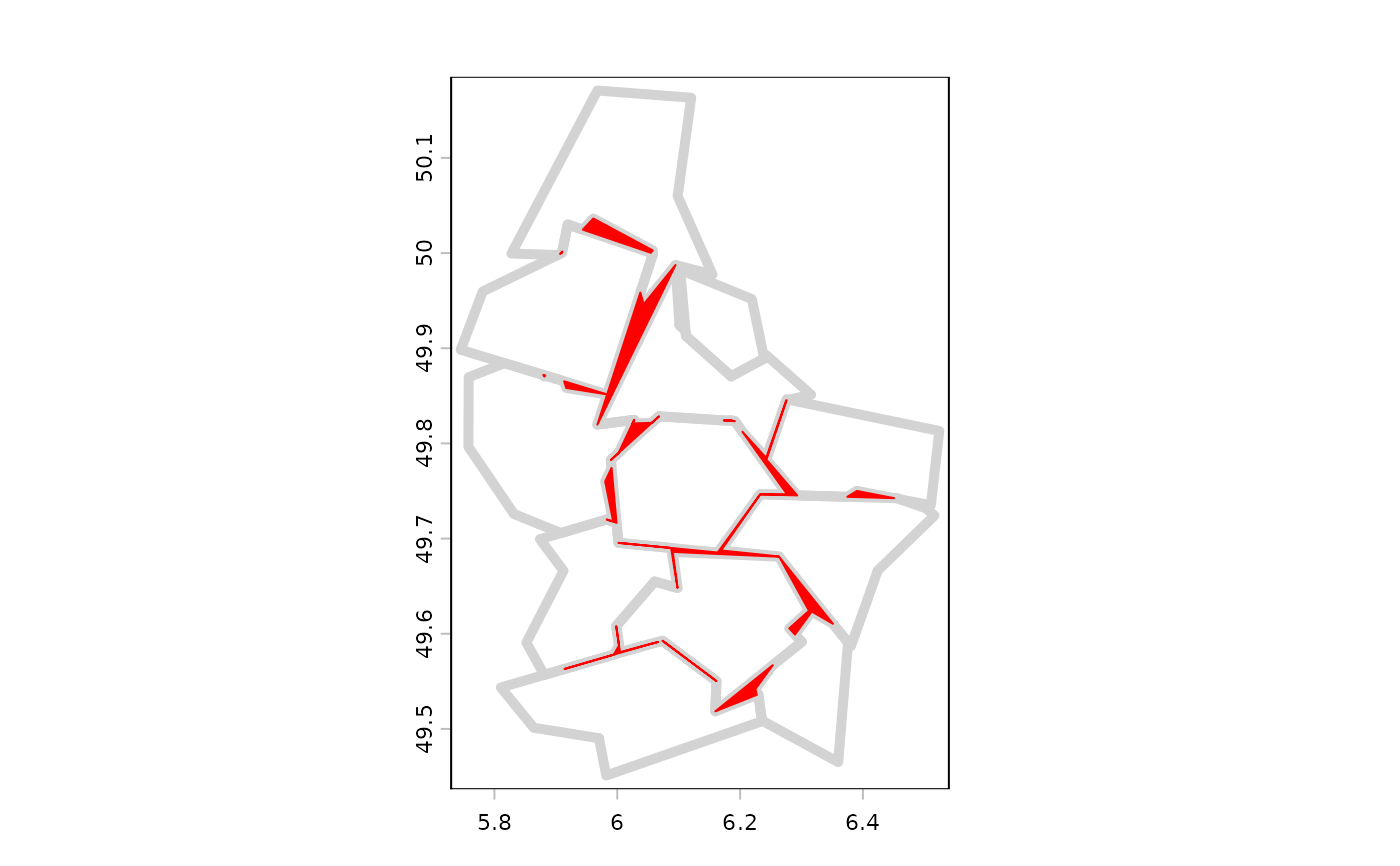Reduce the number of nodes used to represent geometries.

# S4 method for SpatVector
simplifyGeom(x, tolerance=0.1, preserveTopology=TRUE, makeValid=TRUE)

## Arguments

x

SpatVector of lines or polygons

tolerance

numeric. The minimum distance between nodes in units of the crs (i.e. degrees for long/lat)

preserveTopology

logical. If TRUE the topology of output geometries is preserved

makeValid

logical. If TRUE, makeValid is run after simplification to assure that the output polygons are valid

## Value

SpatVector

sharedPaths, gaps, is.valid

## Examples

f <- system.file("ex/lux.shp", package="terra")
v <- vect(f)
w <- simplifyGeom(v, .02, makeValid=FALSE)
e <- erase(w)
g <- gaps(e)
plot(e, lwd=5, border="light gray")
polys(g, col="red", border="red")